# Place value Worksheets for Grade 1

Download Place value Worksheets for Grade 1Q.1) ​Count the number of squares and tick the correct option?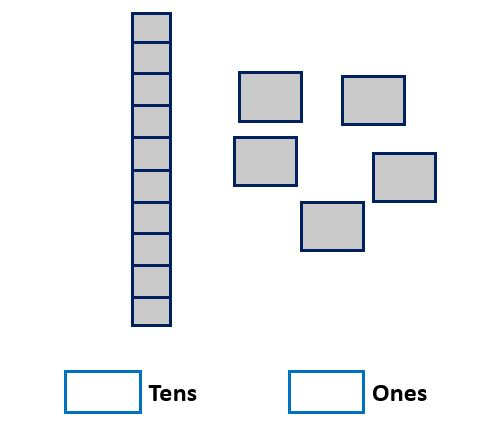[A] ​1 tens 5 ones

[B] ​9 tens 4 ones

Q.2) ​Count the number of squares and tick the correct option?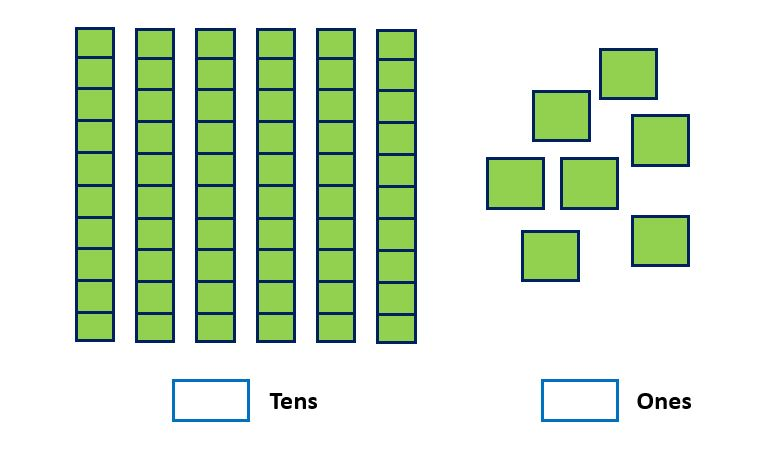[A] ​7 tens 6 ones

[B] ​6 tens 7 ones

Q.3) ​Count the number of squares and tick the correct option?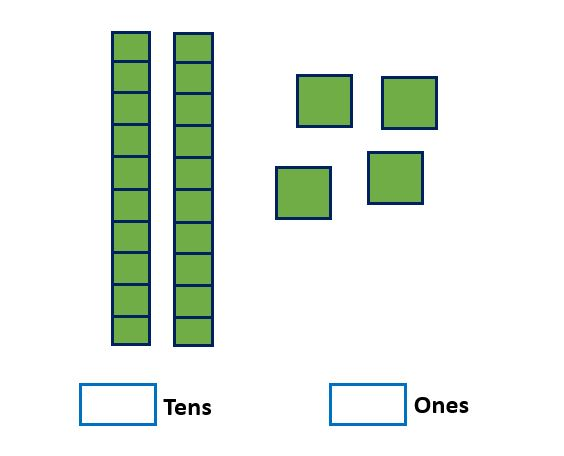[A] ​2 tens 4 ones

[B] ​4 tens 2 ones

Q.4) ​Count the number of squares and tick the correct option?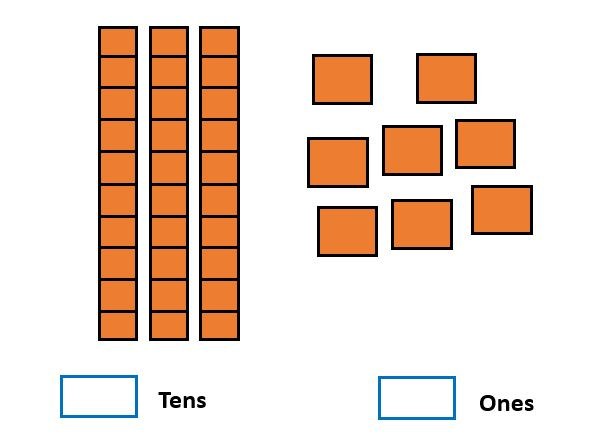[A] ​3 tens 8 ones
[B] ​4 tens 8 ones

Q.5) ​Count the number of squares and tick the correct option?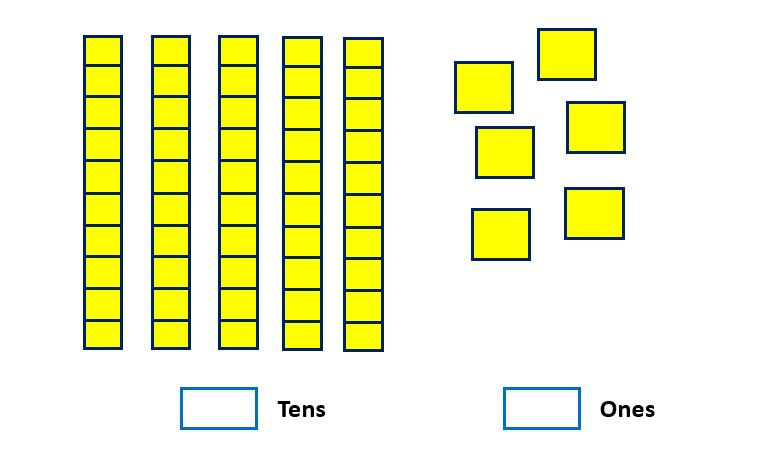[A] ​5 tens 4 ones
[B] ​5 tens 6 ones

Q.6) ​Count the number of squares and tick the correct option?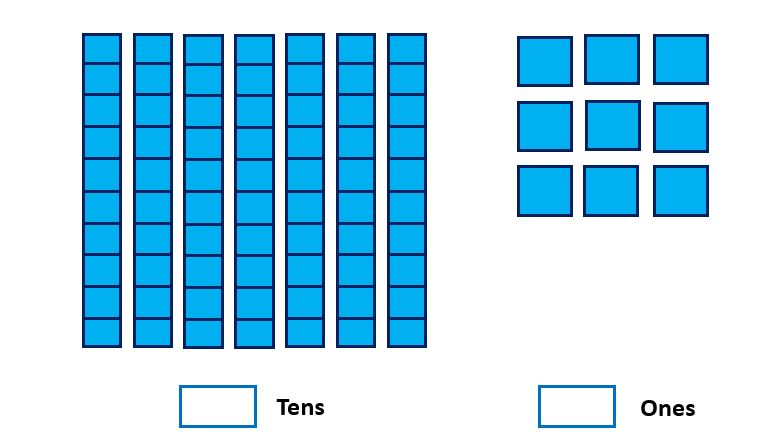​[A] ​7 tens 9 ones
[B] ​8 tens 4 ones

Q.7) ​Count the number of squares and tick the correct option?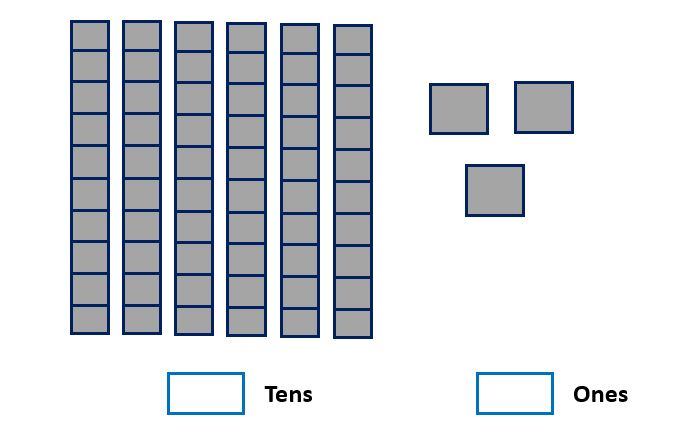​[A] ​4 tens 3 ones
[B] ​6 tens 3 ones

Q.8) ​Count the number of squares and tick the correct option?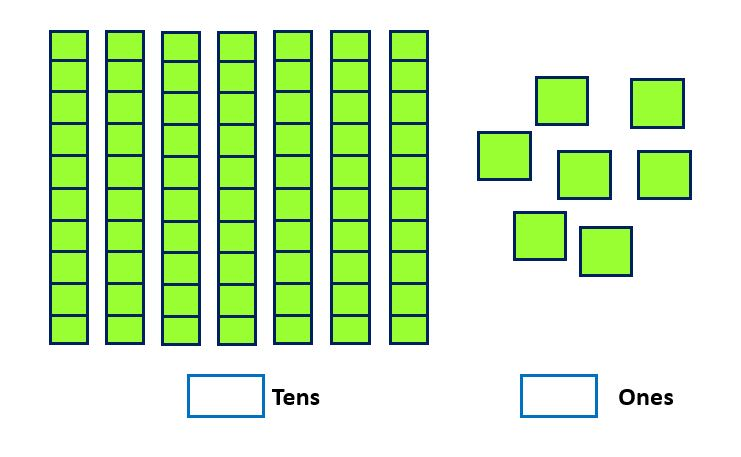[A] ​7 tens 7 ones
[B] ​7 tens 6 ones

Q.9) ​Count the number of squares and tick the correct option?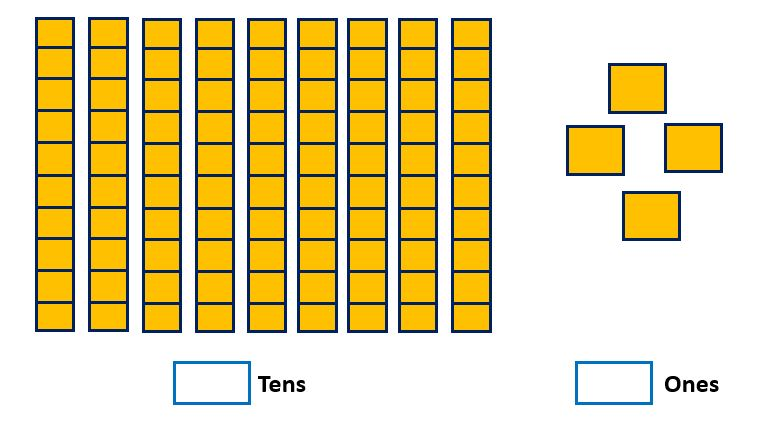​[A] ​9 tens 4 ones
[B] ​8 tens 4 ones

Q.10) ​Count the number of squares and tick the correct option?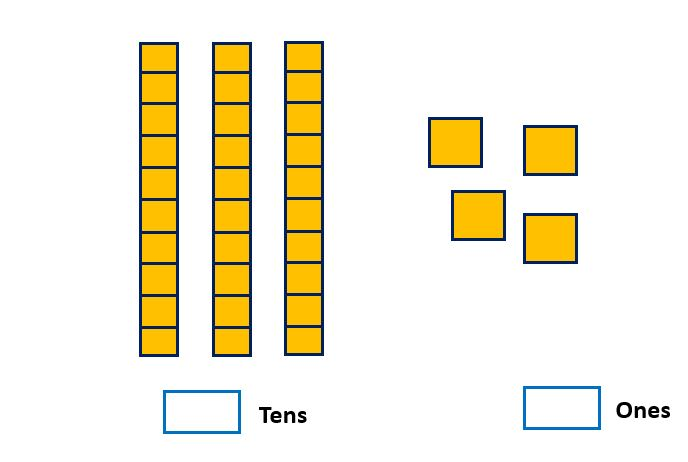[A] ​3 tens 4 ones
[B] ​3 tens 3 ones

Q.1)A
Q.2)B
Q.3)A
Q.4)A
Q.5)B
Q.6)A
Q.7)B
Q.8)A
Q.9)A
Q.10)A